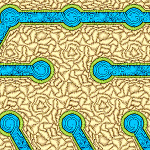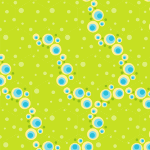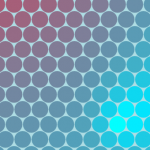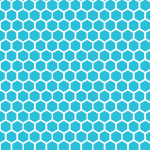The Grids plugin comes with more than 40 examples, which includes simple grid setup, grid algorithms, and grid games. Below are some of the examples you can play on our web site.### Prim’s Maze Generation Algorithm on a Triangular Grid

This example shows how to work with grid edges, and how you can use rhombille grids  to display the edges of triangular grids nicely.### Prim’s Maze Generation Algorithm on a Hexagonal Grid

This example shows how faces and edges of a hex grid can be represented using a single hex grid.### Diffusion

This example shows how to use the AggregateNeighborhood function, in this case, to implement diffusion. In games, diffusion-type algorithms is useful to simulate the flow of gases, disease, and information.### 250 000 Hexes

This example shows how well grids work with big grids.

### Full list of examples

Grids with code

• Start here
• Pointy Hex
• Rect
• Basic grids
• Cario
• Diamond
• Flat Hex
• Flat Rhomb
• Flat Tri
• Pointy Rhomb
• Pointy Tri
• Brick grids
• Flat Brick
• Pointy Brick
• Polar Grids
• Polar Flat Brick
• Polar Pointy Brick
• Polar Rect
• Advanced Grids
• Arhcimedean Spiral
• Generic Spliced
• Layered
• Nested
• Point List
• Advanced Maps
• Image Map
• Stress
• Games
• Block Slider

#### Grids in the Editor

• Start here
• All grids
• 3D
• Hex
• Rect
• Algorithms
• Tiles as edges
• Pointy Rhomb Hex Edge
• Pointy Romb Tri Edge
• Advanced Setup
• Animation
• Shape Building
• Games
• Lights Out on Cairo
• Lights Out on Hex
• Lights Out on Tri
• Lines
• Pipes
• Slider Puzzle